NCERT Exemplar Class 11 Maths Chapter 9 Sequence and Series are part of NCERT Exemplar Class 11 Maths. Here we have given NCERT Exemplar Class 11 Maths Chapter 9 Sequence and Series.

## NCERT Exemplar Class 11 Maths Chapter 9 Sequence and Series

Q1. The first term of an A.P. is terms is zero, show that the sum of its next q terms
$$\frac { -a(p+q)q }{ p-1 }$$

Sol: Let the common differeence of the given A.P be d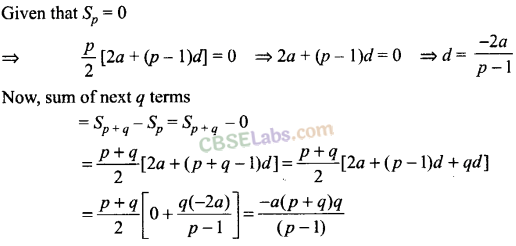Q2. A man saved Rs. 66000 in 20 years. In each succeeding year after the first year, he saved Rs. 200 more than what he saved in the previous year. How much did he save in the first year?
Sol: Let us assume that the man saved Rs.a in the first year.
In each succeeding year, an increment of Rs. 200 is made. So, it forms an A.P. whose
First term = a, Common difference, d = 200 and n=20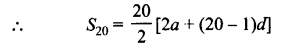=> 6600 = 2a + 19 x 200 => 2a = 2800
∴a = 1400

Q3. A man accepts a position with an initial salary of Rs. 5200 per month. It is understood that he will receive an automatic increase of Rs. 320 in the very next month and each month thereafter.
(i) Find his salary for the tenth month.
(ii) What is his total earnings during the first year?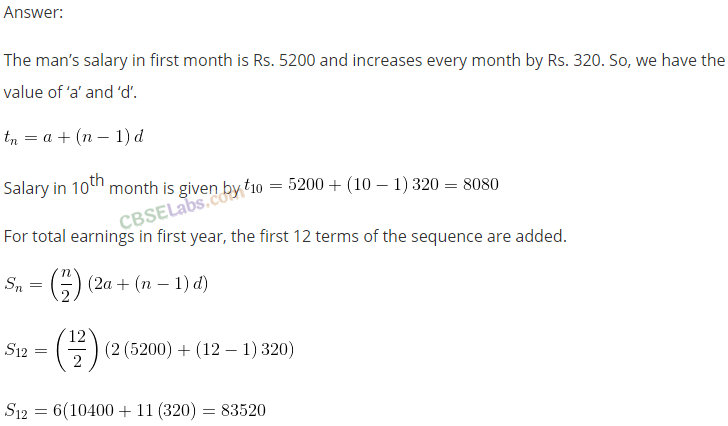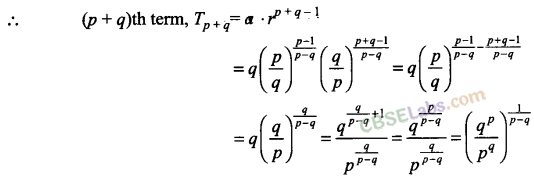Q5. A carpenter was hired to build 192 window frames. The first day he made five frames and each day, thereafter he made two more frames than he made the day before. How many days did it take him to finish the job?
Sol: Here, a = 5 and d = 2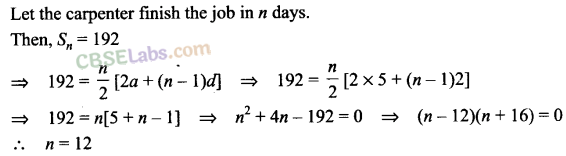Q6. The sum of interior angles of a triangle is 180°. Show that the sum of the interior angles of polygons with 3, 4, 5, 6, … sides form an arithmetic progression. Find the sum of the interior angles for a 21 sided polygon.
Sol: We know that, sum of interior angles of a polygon of side n is (n – 2) x 180°.Q7. A side of an equilateral triangle is 20 cm long. A second equilateral triangle is inscribed in it by joining the mid-points of the sides of the first triangle. This process is continued for third, fourth, fifth, triangles. Find the perimeter of the sixth inscribed equilateral triangle.
Sol: Let the given equilateral triangle be ∆ ABC with each side of 20 cm.
By joining the mid-points of this triangle, we get another equilateral triangle of side equal to half of the length of side of ∆ABC.
Continuing in this way, we get a set of equilateral triangles with side equal to half of the side of the previous triangle.
Now,
Perimeter of first triangle = 20 x 3 = 60 cm;
Perimeter of second triangle = 10 x 3 = 30 cm;
Perimeter of third triangle = 5×3 = 15 cm;Q8. In a potato race 20 potatoes are placed in a line at intervals of 4 m with the first potato 24 m from the starting point. A contestant is required to bring the potatoes back to the starting place one at a time. How far would he run in bringing back all the potatoes?
Sol: Distance travelled to bring first potato = 24 + 24 = 2 x 24 = 48 m
Distance travelled to bring second potato = 2(24 + 4) = 2 x 28 = 56 m
Distance travelled to bring third potato = 2(24 + 4 + 4) = 2 X 32 = 64 m; and so on…
Clearly, 48, 56, 64,… is an A.P. with first term 48 and common difference 8. Also, number of terms is 20.
Total distance run in bringing back all the potatoes,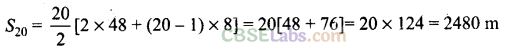Q9. In a cricket tournament 16 school teams participated. A sum of Rs. 8000 is to be awarded among themselves as prize money. If the last placed team is awarded Rs. 275 in prize money and the award increases by the same amount for successive finishing places, how much amount will the first place team receive?
Sol: Let the first place team get Rs. a as the prize money.
Since award money increases by the same amount for successive finishing places, we get an A.P.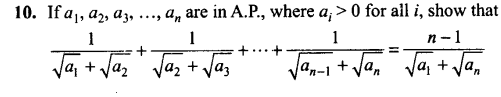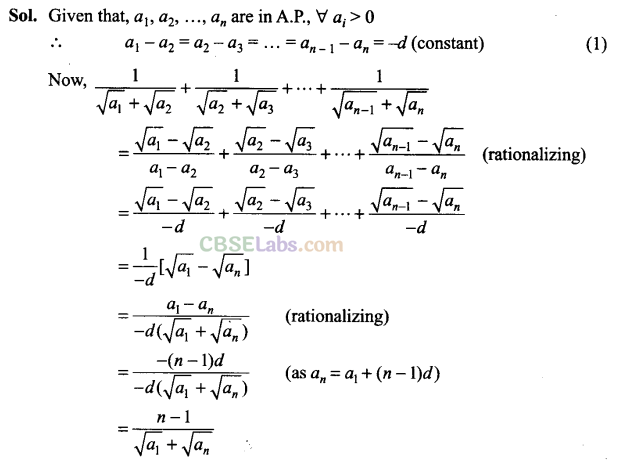Q11. Find the sum of the series (33 – 23) + (53 – 43) + (73 – 63) + … to
(i) n terms
(ii) 10 terms
Sol: Given series is: (33 — 23) + (53 – 43) + (73 – 63) + … n terms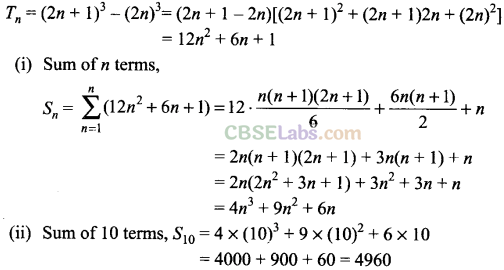Q12. Find the rth term of an A.P. sum of whose first n terms is 2n +3n2
Sol:
Sum of k terms of A.P., Sn = 2n + 3n2Q13. If A is the arithmetic mean and G1 , G2 be two geometric means between any two numbers, then prove that 2A = $$\frac { { G }_{ 1 }^{ 2 } }{ { G }_{ 2 } } +\frac { { G }_{ 2 }^{ 2 } }{ { G }_{ 2 } } \quad$$
Sol: Let the numbers be a and b.Q15. 1f the sum of p terms of an AP. is q and the sum of q terms isp, then show that the sum ofp + q terms is —(p + q). Also, find the sum of first p — q terms (where, p > q).
Sol:
Let first term and common difference of the A.P. be a and d, respectively. Given, Sp = qQ16. If pth, qth and rth terms of an A.P. and G.P. are both a, b and c, respectively, then show that ab-c bc-a-ca-b = 1.
Sol:
Let A and d be the first term and common difference of A.P., respectively. Also, let B and R be the first term and common ratio of G.P., respectively.
It is given that,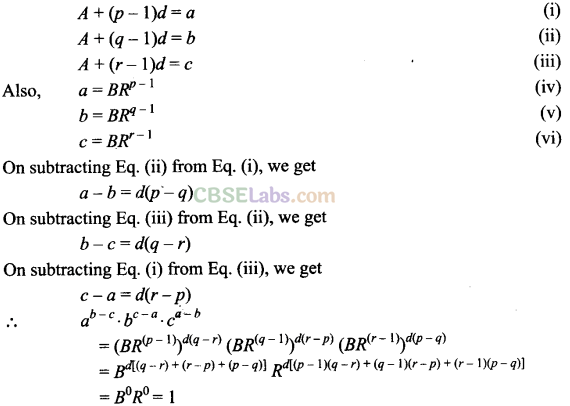Objective Type Questions:

Q17. If the sum of n terms of an A.P. is given by Sn = 3n + 2n2, then the common difference of the A.P. is
(a) 3
(b) 2
(c) 6
(d) 4
Sol: (d) Given, Sn = 3n + 2n2Q18. If the third term of G.P. is 4, then the product of its first 5 terms is
(a) 43
(b) 44
(c) 45
(d) none of these

Sol: (c) Let a and r the first term and common ratio, respectively.Q19. If 9 times the 9th term of an A.P. is equal to 13 times the 13th term, then the 22nd term of the A.P. is
(a) 0
(b) 22
(c) 198
(d) 220
Sol:
(a) Let the first term and coiqmon difference of given A.P. be a and d, respectively.Q20. If x, 2y and 3z are in A.P. where the distinct numbers x, y and z are in G.P., then the common ratio of the G.P.is
Sol:
Since x, 2y and 3z are in A.P., we get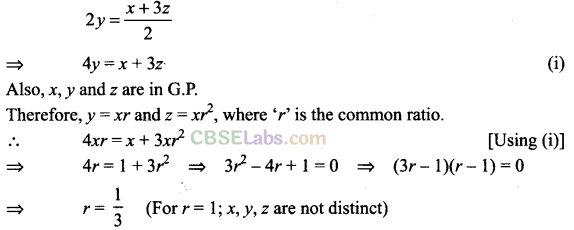Q21. If in an A.P., Sn = qn2 and Sm = qm2, where Sr denotes the sum of r terms of the AP, then Sq equalsQ22. Let Sn denote the sum of the first n terms of an A.P. If S2n = 3Sn, then S3n : Sn  is equal to
(a) 4
(b) 6
(c) 8
(d) 10
Sol:
(b) Let first term be a and common difference be d.
Then, S2n = 3Sn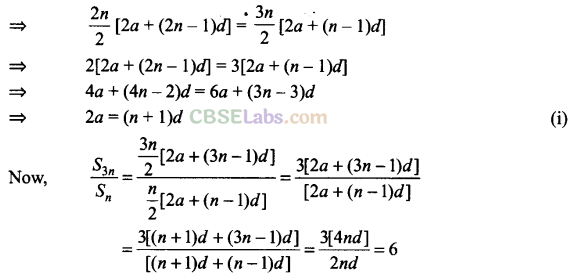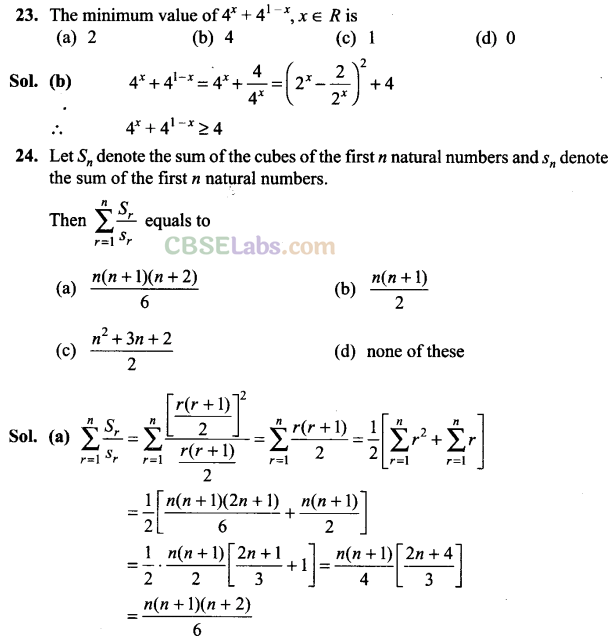Q25. If tn denotes the nth term of the series 2 + 3+ 6+11 + 18+…, then t50 is
(a) 492 – 1
(b) 492
(c) 502+l
(d) 492 +2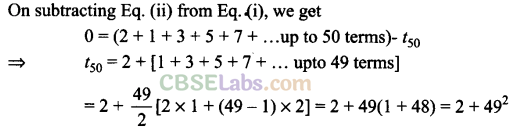Q26. The lengths of three unequal edges of a rectangular solid block are in G.P. If the volume of the block is 216 cm3 and the total surface area is 252 cm2, then the length of the longest edge is
(a) 12 cm

(b) 6 cm
(c) 18 cm
(d) 3 cm
Sol: (a) Let the length, breadth and height of rectangular solid block be a/r, a and ar, respectively.Fill in the Blanks

Q27. If a, b and c are in G.P., then the value of $$\frac { a-b }{ b-c }$$ is equal to ________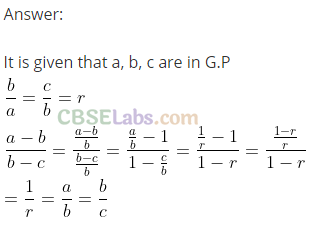Q28. The sum of terms equidistant from the beginning and end in an A.P. is equal to _____.
Sol:
Let a be the first term and d be ihe common difference of the A.P.Q29. The third term of a G.P. is 4. The product of the first five terms is
Sol: Let a and r the first term and common ratio, respectively.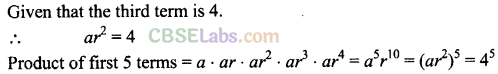True/False Type Questions

Q30. Two sequences cannot be in both A.P. and G.P. together.
Sol: False
Consider the sequence 3,3,3; which is A.P. and G.P. both.

Q31. Every progression is a sequence but the converse, i.e., every sequence is also a progression need not necessarily be true.
Sol: True                                                            –
Consider the progression a, a + d, a + 2d, … and sequence of prime number 2, 3, 5, 7, 11,…
Clearly, progression is a sequence but sequence is not progression because it does not follow a specific pattern.

Q32. Any term of an A.P. (except first) is equal to half the sum of terms which are equidistant from it.
Sol: True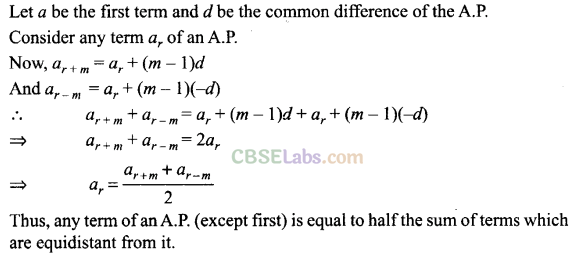Q33. The sum or difference of two G.P.s, is again a G.P.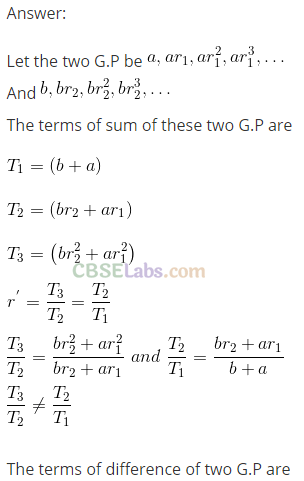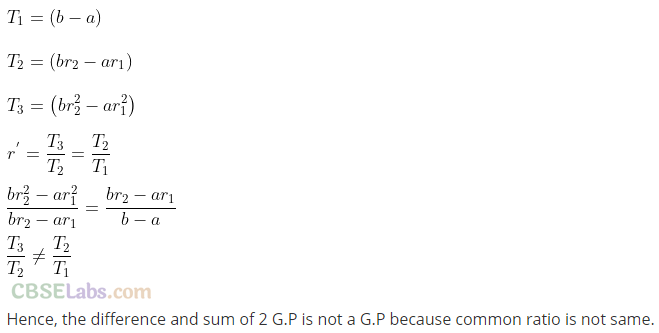Q34. If the sum of n terms of a sequence is quadratic expression, then it always represents an A.P.
Sol: False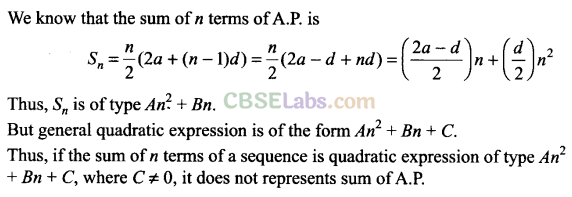Match the questions given under Column I with their appropriate answers given under the column II.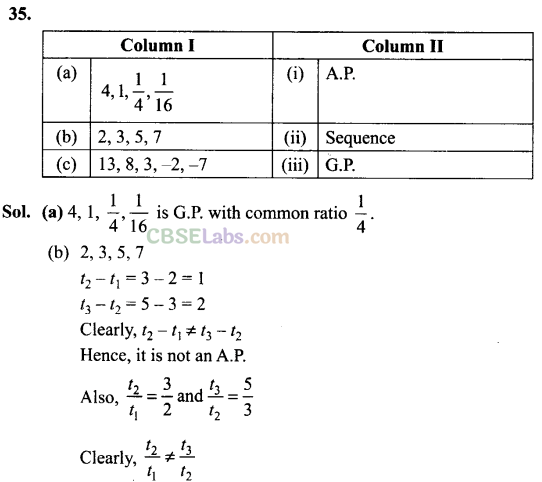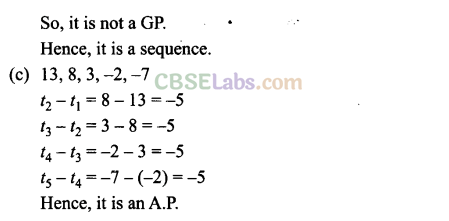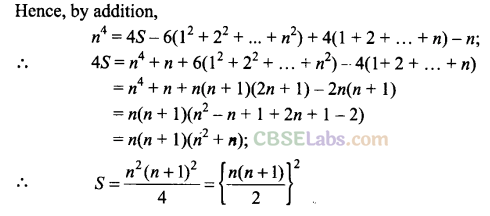We hope the NCERT Exemplar Class 11 Maths Chapter 9 Sequence and Series help you. If you have any query regarding NCERT Exemplar Class 11 Maths Chapter 9 Sequence and Series, drop a comment below and we will get back to you at the earliest.